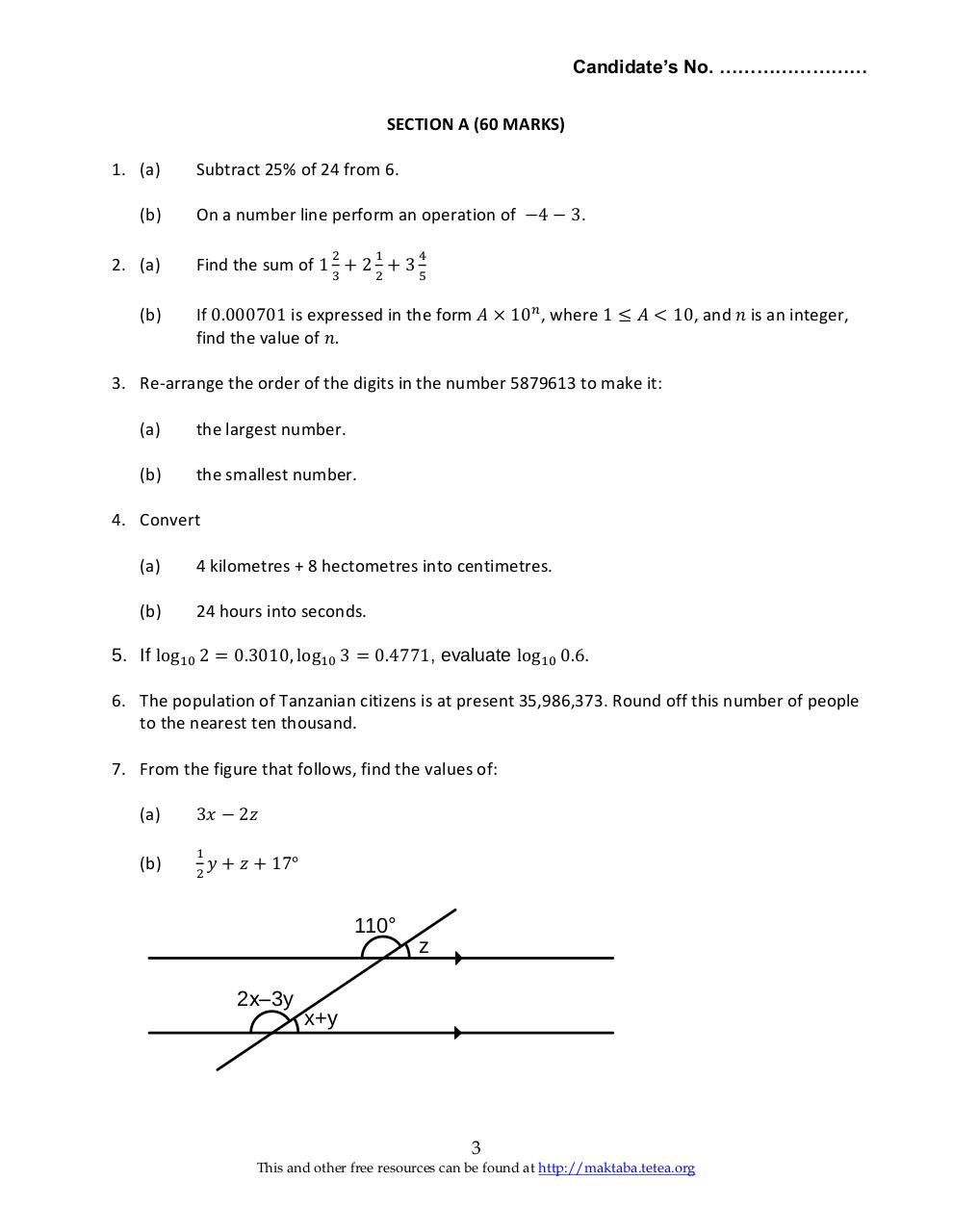# Basic Math 2005 F2.pdfPage 1 2 3 4 5 6

#### Text preview

Candidate’s No. ……………………
SECTION A (60 MARKS)
1. (a)
(b)
2. (a)
(b)

Subtract 25% of 24 from 6.
On a number line perform an operation of −4 − 3.
2

1

4

Find the sum of 1 3 + 2 2 + 3 5
If 0.000701 is expressed in the form 𝐴 × 10𝑛 , where 1 ≤ 𝐴 &lt; 10, and 𝑛 is an integer,
find the value of 𝑛.

3. Re-arrange the order of the digits in the number 5879613 to make it:
(a)

the largest number.

(b)

the smallest number.

4. Convert
(a)

4 kilometres + 8 hectometres into centimetres.

(b)

24 hours into seconds.

5. If log10 2 = 0.3010, log10 3 = 0.4771, evaluate log10 0.6.
6. The population of Tanzanian citizens is at present 35,986,373. Round off this number of people
to the nearest ten thousand.
7. From the figure that follows, find the values of:
(a)
(b)

3𝑥 − 2𝑧
1
2

𝑦 + 𝑧 + 17°

110°

2x–3y

z

x+y

3
This and other free resources can be found at http://maktaba.tetea.org